# Difference between revisions of "Hyperbolic functions"

The functions given by the formulas: \begin{equation} \sinh x = \frac{e^x-e^{-x}}{2}, \end{equation} the hyperbolic sine; and \begin{equation} \cosh x = \frac{e^x+e^{-x}}{2}, \end{equation} the hyperbolic cosine. The hyperbolic tangent \begin{equation} \tanh x = \frac{\sinh x}{\cosh x}, \end{equation} is also sometimes considered. Other notations include: $\operatorname{sh} x$, $\operatorname{Sh} x$, $\operatorname{ch} x$, $\operatorname{Ch} x$, $\operatorname{tgh} x$, $\operatorname{th} x$, $\operatorname{Th} x$. The graphs of these functions are shown in Fig. a.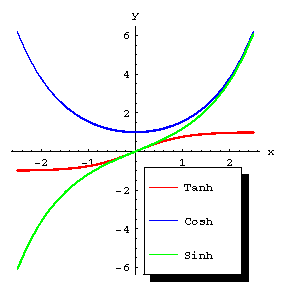Figure: h048250a

The principal relations are: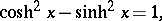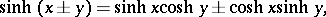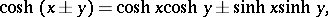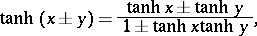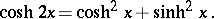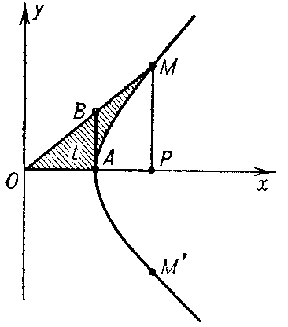Figure: h048250b

The geometrical interpretation of hyperbolic functions is similar to that of the trigonometric functions (Fig. b). The parametric equations of hyperbolas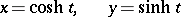make it possible to interpret the abscissa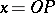and the ordinateof a pointon the equilateral hyperbolaas the hyperbolic sine and cosine; the hyperbolic tangent is the segment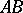. The parameterof the pointequals twice the area of the sector, whereis the arc of the hyperbola. The parameteris negative for a point(for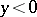).

The inverse hyperbolic functions are defined by the formulas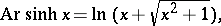The derivatives and basic integrals of the hyperbolic functions are: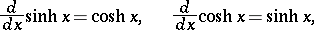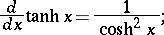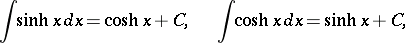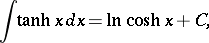The hyperbolic functionsandmay also be defined by the series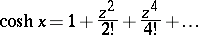in the entire complex-plane, so that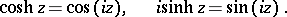(3)

Extensive tabulated values of hyperbolic functions are available. The values of the hyperbolic functions may also be obtained from tables giving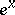and.